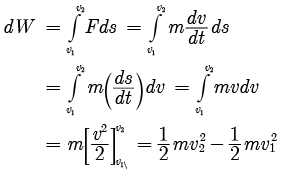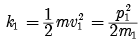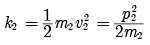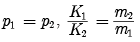Test: Work and Energy- Assertion & Reason Type Questions- 2

# Test: Work and Energy- Assertion & Reason Type Questions- 2 - Class 9

Test Description

## 15 Questions MCQ Test Science Class 9 - Test: Work and Energy- Assertion & Reason Type Questions- 2

Test: Work and Energy- Assertion & Reason Type Questions- 2 for Class 9 2023 is part of Science Class 9 preparation. The Test: Work and Energy- Assertion & Reason Type Questions- 2 questions and answers have been prepared according to the Class 9 exam syllabus.The Test: Work and Energy- Assertion & Reason Type Questions- 2 MCQs are made for Class 9 2023 Exam. Find important definitions, questions, notes, meanings, examples, exercises, MCQs and online tests for Test: Work and Energy- Assertion & Reason Type Questions- 2 below.
Solutions of Test: Work and Energy- Assertion & Reason Type Questions- 2 questions in English are available as part of our Science Class 9 for Class 9 & Test: Work and Energy- Assertion & Reason Type Questions- 2 solutions in Hindi for Science Class 9 course. Download more important topics, notes, lectures and mock test series for Class 9 Exam by signing up for free. Attempt Test: Work and Energy- Assertion & Reason Type Questions- 2 | 15 questions in 30 minutes | Mock test for Class 9 preparation | Free important questions MCQ to study Science Class 9 for Class 9 Exam | Download free PDF with solutions
 1 Crore+ students have signed up on EduRev. Have you?
Test: Work and Energy- Assertion & Reason Type Questions- 2 - Question 1

### Direction: In the following questions, a statement of assertion is followed by a statement of reason. Mark the correct choice as:Assertion : Work done by or against gravitational force in moving a body from one point to another is independent of the actual path followed between the two points.Reason : Gravitational forces are conservative forces.

Detailed Solution for Test: Work and Energy- Assertion & Reason Type Questions- 2 - Question 1
From, definition, work done in moving a body against a conservative force is independent of the path followed.
Test: Work and Energy- Assertion & Reason Type Questions- 2 - Question 2

### Direction: In the following questions, a statement of assertion is followed by a statement of reason. Mark the correct choice as:Assertion : Graph between potential energy of spring versus the extension or compression of the spring is a straight line.Reason : Potential energy of a stretched or compressed spring, is directly proportional to square of extension or compression.

Detailed Solution for Test: Work and Energy- Assertion & Reason Type Questions- 2 - Question 2
Assertion is false but reason is true.

Potential energy (u) = ½(kx2)

i.e. u ∝ x2

This is an equation of parabola, so graph between u and x is a parabola, not straight line.

Test: Work and Energy- Assertion & Reason Type Questions- 2 - Question 3

### Direction: In the following questions, a statement of assertion is followed by a statement of reason. Mark the correct choice as:Assertion : The work done during a round trip is always zero.Reason : No force is required to move a body in its round trip.

Detailed Solution for Test: Work and Energy- Assertion & Reason Type Questions- 2 - Question 3
The work done by a non-conservative force in a round trip is not zero. Since the body moves, there must be force acting on the body.
Test: Work and Energy- Assertion & Reason Type Questions- 2 - Question 4

Direction: In the following questions, a statement of assertion is followed by a statement of reason. Mark the correct choice as:

Assertion : Watt hour has units of energy.

Reason : Kilowatt hour (kW h) is the unit of electric power.

Detailed Solution for Test: Work and Energy- Assertion & Reason Type Questions- 2 - Question 4
Kilowatt (1000 watt) is the unit of power. Kilowatt hour is the power consumed in one hour = power x time = energy. One watt is equal to Joule per second. Hence, watt # time has units of energy.
Test: Work and Energy- Assertion & Reason Type Questions- 2 - Question 5

Direction: In the following questions, a statement of assertion is followed by a statement of reason. Mark the correct choice as:

Assertion : The kinetic energy, with any reference, must be positive.

Reason : In the expression for kinetic energy, the velocity appears with power 2 and mass is a scalar quantity.

Detailed Solution for Test: Work and Energy- Assertion & Reason Type Questions- 2 - Question 5
KE with any reference must be positive as in its expression velocity appears as a square i.e., with power 2 and mass is never negative.
Test: Work and Energy- Assertion & Reason Type Questions- 2 - Question 6

Direction: In the following questions, a statement of assertion is followed by a statement of reason. Mark the correct choice as:

Assertion : The work done during a round trip is always zero.

Reason : No force is required to move a body in its round trip.

Detailed Solution for Test: Work and Energy- Assertion & Reason Type Questions- 2 - Question 6
This is typically true only in the special case of conservative force work but need not be true if F is not conservative.
Test: Work and Energy- Assertion & Reason Type Questions- 2 - Question 7

Direction: In the following questions, a statement of assertion is followed by a statement of reason. Mark the correct choice as:

Assertion : The change in kinetic energy of a particle is equal to the work done on it by the net force.

Reason : Change in kinetic energy of particle is equal to the work done only in case of a system of one particle.

Detailed Solution for Test: Work and Energy- Assertion & Reason Type Questions- 2 - Question 7
Work done on the body in order to increase its velocity v1 to v2 is given by,Thus, work done by a force acting on a body is equal to change in kinetic energy of the body. This is true for a system containing any number of particles.

Test: Work and Energy- Assertion & Reason Type Questions- 2 - Question 8

Direction: In the following questions, a statement of assertion is followed by a statement of reason. Mark the correct choice as:

Assertion : A crane P lifts a car upto a certain height in 1 min. Another crane Q lifts the same car upto the same height in 2 min. Then crane P consumes two times more fuel than crane Q.

Reason : Crane P supplies two times more power than crane Q.

Detailed Solution for Test: Work and Energy- Assertion & Reason Type Questions- 2 - Question 8
Fuel is actually the power supplied by a mechanical machine when there is conversion of heat energy to mechanical energy. Here, the fuel consumed by the cranes is equal to the power supplied by the cranes.Let the mass of the car be m, g be the acceleration due to gravity and h be the height up to which the car is lifted by the cranes.

For crane P: The work done by the crane P is equal to WP = mgh. The power supplied by the crane will be PP = WP/t = mgh/1 = mgh and the fuel consumed will also be the same.

For crane Q: The work done by the crane Q is equal to WQ = mgh. The power supplied by the crane will be PQ = WQ/t = mgh/2 and the fuel consumed will also be the same.

As you can see that PQ = PP/2 → PP = 2PQ, which means that the crane P consumes two times more fuel than crane Q and also crane P supplies two times more power than crane Q.

Test: Work and Energy- Assertion & Reason Type Questions- 2 - Question 9

Direction: In the following questions, a statement of assertion is followed by a statement of reason. Mark the correct choice as:

Assertion : A light body and heavy body have same momentum. Then they also have same kinetic energy.

Reason : Kinetic energy depends on mass of the body.

Detailed Solution for Test: Work and Energy- Assertion & Reason Type Questions- 2 - Question 9
Kinetic energy of a body of mass m1,Again, kinetic energy of a body of mass m2,IfAs given m2 > m1

Therefore, K1 > K2 i.e. the kinetic energy of light body will be more than the kinetic energy of heavy body when both have same momentum.

Test: Work and Energy- Assertion & Reason Type Questions- 2 - Question 10

Direction: In the following questions, a statement of assertion is followed by a statement of reason. Mark the correct choice as:

Assertion : A spring has potential energy, both when it is compressed or stretched.

Reason : In compressing or stretching, work is done on the spring against the restoring force.

Detailed Solution for Test: Work and Energy- Assertion & Reason Type Questions- 2 - Question 10
The work done on the spring against the restoring force is stored as potential energy in both cases, when it is compressed or stretched.
Test: Work and Energy- Assertion & Reason Type Questions- 2 - Question 11

Direction: In the following questions, a statement of assertion is followed by a statement of reason. Mark the correct choice as:

Assertion : Work done by the gravitational force through a certain distance is constant irrespective of the fact that the body has a uniform or accelerated motion.

Reason : Gravitational force is a conservative force.

Detailed Solution for Test: Work and Energy- Assertion & Reason Type Questions- 2 - Question 11
Work done by the gravitational force in moving a body through a certain distance remains the same whether the body moves uniformly or with decreasing acceleration.It is correct statement.

Gravitational forces are conservative forces.It is correct statement But reason is not correct explanation of assertion.

Test: Work and Energy- Assertion & Reason Type Questions- 2 - Question 12

Direction: In the following questions, a statement of assertion is followed by a statement of reason. Mark the correct choice as:

Assertion : A spring has potential energy, both when it is compressed or stretched.

Reason : In compressing or stretching, work is done on the spring against the restoring force.

Detailed Solution for Test: Work and Energy- Assertion & Reason Type Questions- 2 - Question 12
When spring is compressed or stretched, the work is done on the spring. Due to this work, the energy gets stored in it as elastic potential energy.
Test: Work and Energy- Assertion & Reason Type Questions- 2 - Question 13

Direction: In the following questions, a statement of assertion is followed by a statement of reason. Mark the correct choice as:

Assertion : When the force retards the motion of a body, the work done is negative.

Reason : Work done depends on angle between force and displacement.

Detailed Solution for Test: Work and Energy- Assertion & Reason Type Questions- 2 - Question 13
When the force retards the motion, the force and displacement are in opposite directions to each other. Hence, the work done by the force is negative.
Test: Work and Energy- Assertion & Reason Type Questions- 2 - Question 14

Direction: In the following questions, a statement of assertion is followed by a statement of reason. Mark the correct choice as:

Assertion : The kinetic energy, with any reference, must be positive.

Reason : It is because, in the expression for kinetic energy, the velocity appears with power 2 and mass is a scalar quantity.

Detailed Solution for Test: Work and Energy- Assertion & Reason Type Questions- 2 - Question 14
KE with any reference must be positive as in its expression velocity appears as a square i.e., with power 2 and mass is never negative.
Test: Work and Energy- Assertion & Reason Type Questions- 2 - Question 15

Direction: In the following questions, a statement of assertion is followed by a statement of reason. Mark the correct choice as:

Assertion : According to law of conservation of mechanical energy, change in potential energy is equal and opposite to the change in kinetic energy.

Reason : Mechanical energy is not a conserved quantity.

Detailed Solution for Test: Work and Energy- Assertion & Reason Type Questions- 2 - Question 15
For conservative forces the sum of kinetic and potential energies at any point remains constant throughout the motion. It does not depend upon time. This is known as law of conservation of mechanical energy. According to this rule.

Kinetic energy + Potential energy = E = constant

## Science Class 9

66 videos|355 docs|97 tests
Information about Test: Work and Energy- Assertion & Reason Type Questions- 2 Page
In this test you can find the Exam questions for Test: Work and Energy- Assertion & Reason Type Questions- 2 solved & explained in the simplest way possible. Besides giving Questions and answers for Test: Work and Energy- Assertion & Reason Type Questions- 2, EduRev gives you an ample number of Online tests for practice

## Science Class 9

66 videos|355 docs|97 tests